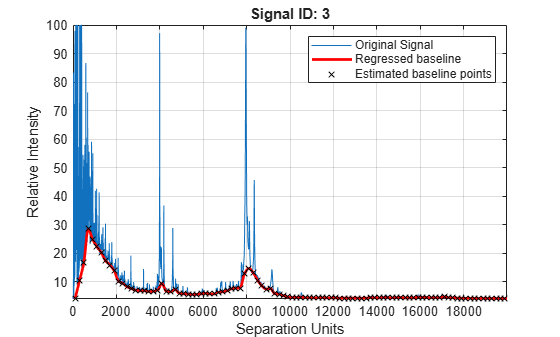Documentation

Correct baseline of signal with peaks

Description

example

yOut = msbackadj(X,Intensities) adjusts the variable baseline of a raw signal with peaks by performing the following steps.

1. Estimate the baseline within multiple shifted windows of width 200 separation units.

2. Regress the varying baseline to the window points using a spline approximation.

3. Adjust the baseline of the peak signals supplied by the input Intensities.

4. Return the adjusted intensity values in the output matrix yOut.

example

yOut = msbackadj(X,Intensities,Name,Value) sets additional options specified by one or more name-value pair arguments. For example, msbackadj(X,Intensities,'WindowSize',300) sets the width of the shifting window to 300 separation units.

Examples

collapse all

Load a sample mass spec data including MZ_lo_res, a vector of m/z values, and Y_lo_res, a matrix of intensity values.

Adjust the baseline of a group of spectrograms and show only the third spectrum and its estimated background.Estimate the baseline for every spectrum in Y_lo_res using an anonymous function to describe an m/z dependent parameter. Then plot the estimated background for the fourth spectrum.

wf = @(mz) 200 + .001 .* mz;Input Arguments

collapse all

Separation-unit values for a set of signals with peaks, specified as a vector.

The number of elements in the vector equals the number of rows in Intensities. The separation unit can quantify wavelength, frequency, distance, time, or m/z ratio depending on the instrument that generates the signal data.

Data Types: double

Intensity values for a set of peaks that share separation-unit range, specified as a numeric matrix.

Each row corresponds to a separation-unit value, and each column corresponds to either a set of signals with peaks or a retention time. The number of rows equals the number of elements in X. The signal data can come from any separation technique, such as spectroscopy, NMR, electrophoresis, chromatography, or mass spectrometry.

Data Types: double

Name-Value Pair Arguments

Specify optional comma-separated pairs of Name,Value arguments. Name is the argument name and Value is the corresponding value. Name must appear inside quotes. You can specify several name and value pair arguments in any order as Name1,Value1,...,NameN,ValueN.

Example:

Shifting window size, specified as a positive scalar or function handle. By default, msbackadj estimates baseline points for windows with a width of 200 separation units.

If you specify a function handle, the function is evaluated at the respective X values and returns a variable width for the window. Specifying a function handle is useful when the resolution of the signal is dissimilar at different regions.

The result of msbackadj depends on the window size and step size. Define the parameters based on the width of your peaks in the signal and the presence of possible drifts. If you have wider peaks towards the end of the signal, consider using variable window sizes and/or step sizes.

Example: 'WindowSize',300

Data Types: double | function_handle

Step size for the shifting window, specified as a positive scalar or function handle. By default, msbackadj estimates baseline points for windows placed every 200 separation units.

If you specify a function handle, the function is evaluated at the respective separation-unit values and returns the distance between adjacent windows.

Example: 'StepSize',150

Data Types: double | function_handle

Method to regress the window estimated points to a soft curve, specified as one of the following:

• 'pchip' — Shape-preserving piecewise cubic interpolation. The interpolated value at a query point is based on a shape-preserving piecewise cubic interpolation of the values at neighboring grid points.

• 'linear' — Linear interpolation. The interpolated value at a query point is based on linear interpolation of the values at neighboring grid points in each respective dimension.

• 'spline' — Spline interpolation. The interpolated value at a query point is based on a cubic interpolation of the values at neighboring grid points in each respective dimension.

Example: 'RegressionMethod','linear'

Data Types: char | string

Method to find likely baseline (background) value in every window, specified as one of the following:

• 'quantile' — Quantile value is set to 10%.

• 'em' — Every sample is the independent and identically distributed (i.i.d) draw of any of two normal distributed classes (background or peaks). Because the class label is hidden, the distributions are estimated with an Expectation-Maximization algorithm. The ultimate baseline value is the mean of the background class.

Example: 'EstimationMethod','em'

Data Types: char | string

Method to smooth the curve of estimated points, specified as one of the following:

• 'none' — No smoothing.

• 'lowess' — Linear fit.

• 'loess' — Quadratic fit.

• 'rlowess' — Robust linear fit.

• 'rloess' — Robust quadratic fit.

Example: 'SmoothMethod','lowess'

Data Types: char | string

Quantile value, specified as a positive scalar between 0 and 1.

Example: 'QuantileValue',0.2

Data Types: double

Flag to preserve the height of the tallest peak in the signal, specified as true or false. By default, peak heights are not preserved.

Example: 'PreserveHeights',true

Data Types: logical

Flag to plot the regressed baseline, original signal, and estimated baseline points, specified as true, false, or a positive integer.

The default behavior is as follows:

• When you call msbackadj without an output argument, the plot is shown. Only the first signal from the input Intensities is plotted.

• When you call msbackadj with an output argument, the plot is not shown. But you can get the plot by also setting 'ShowPlot' to true.

You can also specify an index to one of the signals (columns) in Intensities to show the corresponding plot of that signal.

Example: 'ShowPlot',5

Data Types: double | logical

Output Arguments

collapse all

Adjusted intensity values, returned as a matrix.

Introduced before R2006a

Bioinformatics Toolbox DocumentationGet trial now